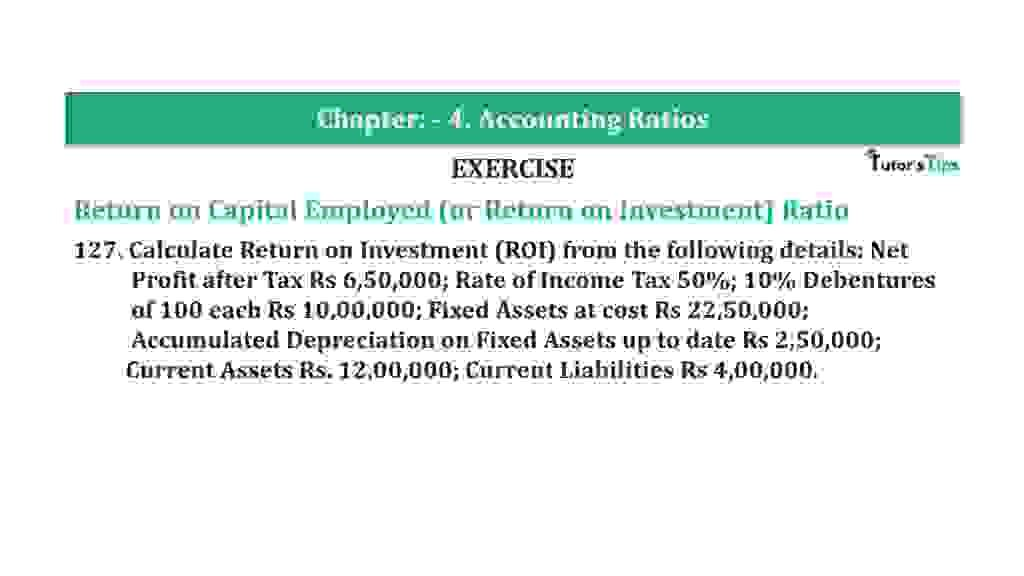# Question 127 Chapter 4 of +2-B – T.S. Grewal 12 ClassQuestion 127 Chapter 4 of +2-B

Return on Capital Employed (or Return on Investment) Ratio

127. Calculate Return on Investment (ROI) from the following details: Net
Profit after Tax Rs 6,50,000; Rate of Income Tax 50%; 10% Debentures
of 100 each Rs 10,00,000; Fixed Assets at cost Rs 22,50,000;
Accumulated Depreciation on Fixed Assets up to date Rs 2,50,000;
Current Assets Rs. 12,00,000; Current Liabilities Rs 4,00,000.

### The solution of Question 127 Chapter 4 of +2-B: –

 Net Fixed Assets = Fixed Assets at cost − Accumulated Depreciation = Rs. 22,50,000 – Rs. 2,50,000 = Rs. 20,00,000 Capital Employed = Net Fixed Assets + Current Assets − Current Liabilities = 20,00,000 + Rs. 12,00,000 – Rs. 4,00,000 = Rs. 28,00,000 Interest of Debentures = Rs. 10,00,000 x 10% = Rs. 1,00,000 Profit before Tax = x Profit after Tax = Profit before Tax − Tax Tax Rate = 50% Tax = .5x x − 0.5 x = Rs. 6,50,000 0.5 x = Rs. 6,50,000
 x = Rs. 6,50,000 0.5 Net Profit before Tax = Rs. 13,00,000

 Profit before Interest and Tax = Profit before Tax + Interest on Long-term Debt = Rs. 14,00,000
 Net Profit Ratio = Profit before Interest and Tax X 100 Capital Employed
 Net Profit Ratio = Rs. 14,00,000 X 100 Rs. 28,00,000 = 50%

Balance Sheet: Meaning, Format & Examples

Thanks, Please Like and share with your friends

Comment if you have any question.

Also, Check out the solved question of previous Chapters: –

### T.S. Grewal’s Double Entry Book Keeping (Vol. II: Accounting for Companies)T.S. Grewal’s Analysis of Financial Statements